# Interactive Demonstrations for Statistics Education on the World Wide Web

R. Webster West and R. Todd Ogden
University of South Carolina

Journal of Statistics Education v.6, n.3 (1998)

Copyright (c) 1998 by R. Webster West and R. Todd Ogden, all rights reserved. This text may be freely shared among individuals, but it may not be republished in any medium without express written consent from the authors and advance notification of the editor.

Key Words: Applets; Central limit theorem; Confidence intervals; Histogram bin width; Influential points; Power; Java; Let's Make a Deal.

## Abstract

The World Wide Web (WWW) is a tool that can be used in many ways for basic statistics education. Using the latest WWW technology, educators can now include interactive demonstrations in the form of Java applets within their WWW materials. Six example applets developed by the authors are introduced and discussed. Suggestions for class use are made, and instructions for incorporating the applets within a WWW document are given.

# 1. Introduction

1 The World Wide Web (WWW) has opened up a new medium for education. There are a number of WWW locations that provide various forms of information for statistics education in electronic form. For example, the Department of Statistics at the University of California-Los Angeles is developing the UCLA Electronic Textbook at

http://www.stat.ucla.edu/textbook/.

The University of Newcastle has also developed the Hyper-Textbook Library at

http://surfstat.newcastle.edu.au/surfstat/

which is a basic statistical text. The Journal of Statistics Education at

http://jse.amstat.org/

is a site dedicated to publishing manuscripts related to statistics education. These WWW sites, as well as numerous other locations offering lecture notes and other course materials, have provided new resources to both students and educators. This access to new perspectives makes the WWW an exciting new forum for statistics education.

2 The information contained at most WWW locations pertaining to statistics education is not significantly different from what one encounters in a classical printed medium. While such information is certainly useful and easily accessible, it is static in that it cannot respond to user input. With the development of the Java computing language (http://java.sun.com/docs/books/tutorial/), it is now possible to add interactive components designed to aid in the understanding of a concept and also stimulate a student's interest by providing a "hands on" learning experience. These interactive components take the form of Java applets that can be shipped over the WWW. The only user requirement is a Java-capable WWW browser such as Netscape Navigator, Microsoft Internet Explorer, or Sun Microsystems' HotJava. It is estimated that over 95% of all people browsing the WWW have such a browser.

3 This paper describes the motivation and usage of six interactive applets written by the authors for the purpose of basic statistics education. These interactive demonstrations were developed to help students in introductory courses better understand some of the more difficult concepts that they encounter. A listing of these applets and other sources of dynamic statistical information may be found at

http://www.stat.sc.edu/rsrch/gasp/.

# 2. Examples of Interactive Applets

## 2.1. Bin Width for Histograms

4 The histogram is one of the first procedures discussed when teaching students graphical techniques for describing data. One important consideration for the construction of a histogram is the choice of the bin width (or equivalently the number of bins) for a given dataset. Choosing too few bins often hides valuable information, and choosing too many bins leads to a messy plot from which little information can be obtained. Many students have a difficult time grasping the effect that bin width has on the shape of a histogram. An applet, located at

http://jse.amstat.org/v6n3/applets/Histogram.html,

allows students to interactively see this effect. A display of the applet as it appears in Netscape Navigator is given in Figure 1. A histogram of the Old Faithful dataset (Azzalini and Bowman 1990) is given when a student loads the applet. Students may interactively change the bin width by clicking and dragging the arrow underneath the bin width scale. The histogram automatically adjusts to the new bin width as the student slides the arrow. The student will quickly see that the bimodal nature of the dataset is hidden when the bin width is too large, and that the histogram reduces to a spike at each data point when the bin width is too small.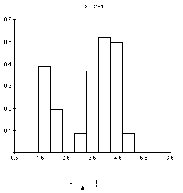Figure 1 (3.3K gif)

Figure 1. Histogram Applet With Old Faithful Data.

## 2.2. Influential Points in Regression

5 One important topic often mentioned when teaching students about basic regression analysis is that extreme observations may have a large effect on the regression line for a particular dataset. An applet, located at

http://jse.amstat.org/v6n3/applets/Regression.html,

interactively shows students the effect of various influential points on regression analysis. A picture of the applet is given in Figure 2. When the applet is loaded, students are given a plot of five data points and the resulting estimated regression line, as well as the equation for the regression line underneath the graphic. By clicking the mouse button, students may add an additional point to the plot. Each time the student adds a point, the new regression line is plotted in red and the equation of the new line is given underneath the original regression line equation. Students are instructed to add points close to the existing regression line and also far from the line. Students will learn from this interactive graphical procedure how individual points can affect the regression line and which outliers exhibit maximal effect.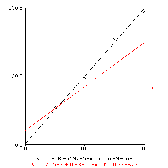Figure 2 (2.7K gif)

Figure 2. Regression Applet.

## 2.3. Central Limit Theorem

6 One concept that students often struggle with is the central limit theorem. To illustrate this concept in a way that would not be feasible without using computing power, the applet located at

http://jse.amstat.org/v6n3/applets/CLT.html

was developed. A picture of the applet is given in Figure 3.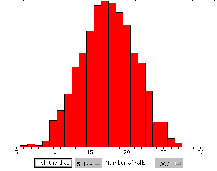Figure 3 (4.8K gif)

Figure 3. Central Limit Theorem Applet.

7 The applet performs a simulation in which a user-specified number of virtual dice can be "rolled." Each time the dice are rolled, an updated histogram of the sum of the dice is constructed. Students can study the way the shape of the histogram changes as more observations are generated. When students get bored repeating the dice-rolling experiment only once for each mouse click, they can select to replicate the experiment more times per mouse click -- up to 10,000 -- so that they can see the convergence in "fast motion."

8 This applet also demonstrates that the central limit theorem applies only when the sample size is relatively large. When students roll a single die repeatedly, the histogram will never get bell-shaped -- it will more and more closely resemble the uniform distribution. Similarly, with two dice, the histogram will tend to resemble a "witch's hat" as the experiment is repeated many times. But for more dice, the histogram will tend to take on the satisfying bell shape, allowing students to see the central limit theorem in action.

## 2.4. Confidence Intervals

9 The first confidence interval encountered in an introductory statistics course is generally a (1 -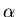) � 100% confidence interval for the mean,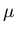, of a normal population with known standard deviation,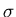.

10 Students can be left with many misconceptions after a standard introductory discussion. One frequent misconception is that a 99% confidence interval is narrower than a 95% confidence interval. Students also tend to misinterpret the confidence interval by considering it to be a fixed quantity, not recognizing its dependence on the particular sample observed. Another common misconception is that the interval is a statement about the distribution of the data, rather than a set of possible "guesses" for the mean of the distribution generating the dataset. This can result from the fact that confidence intervals are generally discussed in reference to a particular dataset rather than a series of datasets.

11 An applet, located at

http://jse.amstat.org/v6n3/applets/ConfidenceInterval.html,

has been designed to help students overcome these misconceptions. A picture of the applet is given in Figure 4.Figure 4 (4.5K gif)

Figure 4. Confidence Interval Applet.

12 When the applet is loaded, confidence intervals for the mean from 50 random samples of size 5 from a standard normal distribution (= 0 and= 1) are generated and plotted along with a horizontal line at the true mean of 0. By clicking and dragging the arrow underneath the "alpha" scale, students may instantaneously change the level of confidence for the intervals. The width of the intervals adjusts automatically aschanges. Students should notice that asgets smaller the intervals get wider. In turn, more intervals will "cover" the true mean of 0 asgets smaller. Intervals that do not cover 0 are colored red so that they are more easily identified. The total number of intervals that cover 0 for a given value ofis also given underneath the graphic.

13 Students may also lock in a value offor a larger simulation study by clicking on the button labeled More Intervals!. At this point, a new set of 50 confidence intervals is displayed at the (1 -) confidence level. Students are free to change the value offor the new set of intervals, but whenever the More Intervals! button is clicked, the default or initially-set-level is used. In addition, a running total of the number of intervals that have covered 0 is given at the bottom of the applet. In repeating this process, students are running a simulation study that can help them understand what a confidence interval really is in terms of the probability of covering the true mean for a given value of. A screen image of the applet as it appears after 1000 simulations with= 0.05 is given in Figure 4.

14 Students may change the defaultby hitting the New Alpha! button, and repeat the simulation study for the newlevel. This process may be repeated several times in order to investigate the coverage probabilities for many differentlevels through simulation.

## 2.5. Power and Hypothesis Testing

15 Students also sometimes wrestle with the concept of the power of a hypothesis test. Although this should be a fairly intuitive concept, students sometimes get bogged down in the mathematical definition of the power of a test. In order to provide students a graphical demonstration of power and to allow them to see how the power of a test changes as the sample size, population standard deviation, and true mean change, the applet located at

http://jse.amstat.org/v6n3/applets/power.html

was developed. A picture of the applet is given in Figure 5.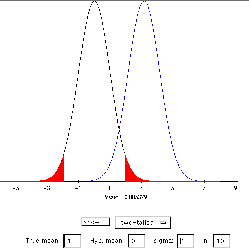Figure 5 (4.9K gif)

Figure 5. Power of a Hypothesis Test Applet.

16 This applet, which deals with the test for the mean of a single population (standard deviationknown), displays both the null distribution (which is always the standard normal) and the "true" distribution of the test statistic Z for the user-supplied "true" value of. The rejection region is shaded in blue under the null distribution (corresponding to area) in red, and the area corresponding to the power is shaded under the "true" distribution. Students may change the population standard deviation, the sample size, the direction of the alternative hypothesis (upper-, lower-, or two-tailed), the hypothesized value for, and the "true" value ofand study how these choices affect the power of the resulting test.

## 2.6. Let's Make a Deal!

17 As a motivating example behind the discussion of probability, an applet has been developed that allows students to investigate the Let's Make a Deal paradox. This paradox is related to a popular television show in the 1970's. In the show scenario, a contestant is given a choice of three doors, one of which hides a prize. The other two doors hide gag gifts like a chicken or a donkey. After the contestant chooses an initial door, the host of the show reveals a gag gift behind one of the two doors not chosen and asks the contestant if he or she would like to switch to the other unchosen door. The question is whether or not the contestant should switch to increase the chances of winning.

18 The intuition of most students tells them that each of the doors, the chosen door and the unchosen door, are equally likely to contain the prize so that there is a 50% probability of winning with either selection, but this is not the case. The probability of winning by using the switching technique is 2/3, while the chance of winning by not switching is 1/3. One way to explain this to students is as follows. The probability of picking the wrong door in the initial stage of the game is 2/3. If the contestant picks the wrong door initially, the host must reveal the remaining empty door in the second stage of the game. Thus, if the contestant switches after picking the wrong door initially, the contestant will win the prize. The probability of winning by switching then reduces to the probability of picking the wrong door in the initial stage, which is clearly 2/3.

19 Despite a very clear explanation of this paradox, most students have difficulty understanding the problem. It is very difficult to overcome the strong intuition that most students have in this case. As a challenge to students who don't believe the explanation, an instructor may ask the students to actually play the game a number of times by switching and by not switching and to keep track of the relative frequency of wins with each strategy. An applet has been developed that allows students to repeatedly play the game and keep track of the results. The WWW address of the applet is

20 Within the applet, the computer plays the role of the host. Upon loading the applet, students are asked to pick a door by clicking the mouse on the proper region. After the initial pick, the computer reveals one of the two gag gifts by "opening" a door and displaying a picture of a donkey behind one of the numbers. The students then have the option of staying with their initial selection or switching to the remaining door. The computer keeps track of the number of times the game is played with each strategy and the number of times the game is won with each strategy. This information is displayed at the bottom of the applet after each game. A screen image of the applet after thirty plays with each strategy is given in Figure 6. Using empirical techniques, the student is then able to see the strategy with the highest probability of winning as the relative frequencies converge to the true probabilities. An instructor may use this game to motivate the idea of repeated trials as a means for investigating a random phenomenon and estimating probabilities.Figure 6 (9.2K gif)

Figure 6. Let's Make a Deal Applet.

# 3. Discussion

21 The authors have experience using the applets described above as in-class demonstrations and for follow-up student assignments. After the relevant concept has been introduced and discussed in class, the applet is demonstrated and its pertinence to the concept is explained. Students are then asked to investigate the applet further on their own time and to answer a set of questions designed to guide their exploration of the concept. Based on student feedback, this learning format has worked very well for a wide range of students.

22 Interested instructors may easily incorporate the example applets into their own WWW material. For example, to include the Central Limit Theorem applet within an HTML document one uses the following applet tag:

      <APPLET
codebase="http://jse.amstat.org/v6n3/applets/classes"
code="CLT.class" width=450 height=400> </APPLET>


The discussion surrounding the applet and the instructions for students may then be customized according to individual instructor preferences. It is also possible to use other data with the histogram and regression applets. To do this, one includes a parameter statement within the applet tag like the following:

      <APPLET
codebase="http://jse.amstat.org/v6n3/applets/classes"
code="Regression.class" width=400 height=400>
<PARAM name=data value="
0.0 0.53
50.0 55.13
100.0 97.9
150.0 155.94
200.0 195.02
">
</APPLET>


The authors have constructed the applets for free public use, but ask that anyone who uses an applet also includes the proper acknowledgment.

23 The authors hope that other educators will find the example applets useful for their own classes. These interactive demonstrations can help statistics educators take advantage of the full capabilities of the WWW. A related project currently under development by the authors is WebStat, an environment for statistical analysis available freely over the WWW. The WWW location of WebStat is

http://www.stat.sc.edu/~west/webstat/.

Soon students will have access not only to the interactive examples discussed in this paper, but also to a complete package for data analysis.

# Reference

Azzalini, A., and Bowman, A. W. (1990), "A Look at Some Data on the Old Faithful Geyser," Applied Statistics, 39, 357-365.

R. Webster West west@stat.sc.edu
R. Todd Ogden ogden@stat.sc.edu
Department of Statistics
University of South Carolina
Columbia, SC 29208